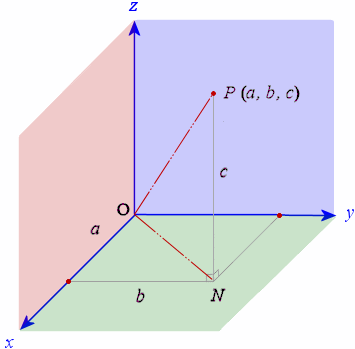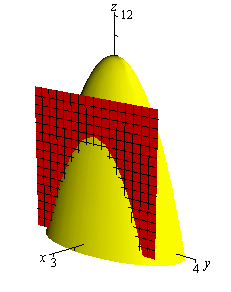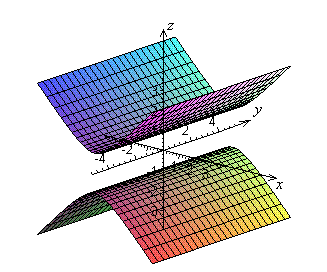Home

#### The Fundamental Theorem of Calculus

Three Different Concepts
The Fundamental Theorem of Calculus (Part 2)
The Fundamental Theorem of Calculus (Part 1)
More FTC 1

#### The Indefinite Integral and the Net Change

Indefinite Integrals and Anti-derivatives
A Table of Common Anti-derivatives
The Net Change Theorem
The NCT and Public Policy

#### Substitution

Substitution for Indefinite Integrals
Examples to Try
Revised Table of Integrals
Substitution for Definite Integrals
Examples

#### Area Between Curves

Computation Using Integration
To Compute a Bulk Quantity
The Area Between Two Curves
Horizontal Slicing
Summary

#### Volumes

Slicing and Dicing Solids
Solids of Revolution 1: Disks
Solids of Revolution 2: Washers
More Practice

#### Integration by Parts

Integration by Parts
Examples
Integration by Parts with a definite integral
Going in Circles

#### Integrals of Trig Functions

Antiderivatives of Basic Trigonometric Functions
Product of Sines and Cosines (mixed even and odd powers or only odd powers)
Product of Sines and Cosines (only even powers)
Product of Secants and Tangents
Other Cases

#### Trig Substitutions

How Trig Substitution Works
Summary of trig substitution options
Examples
Completing the Square

#### Partial Fractions

Introduction
Linear Factors
Improper Rational Functions and Long Division
Summary

#### Strategies of Integration

Substitution
Integration by Parts
Trig Integrals
Trig Substitutions
Partial Fractions

#### Improper Integrals

Type 1 - Improper Integrals with Infinite Intervals of Integration
Type 2 - Improper Integrals with Discontinuous Integrands
Comparison Tests for Convergence

#### Differential Equations

Introduction
Separable Equations
Mixing and Dilution

#### Models of Growth

Exponential Growth and Decay
Logistic Growth

#### Infinite Sequences

Examples of Infinite Sequences
Limit Laws for Sequences
Theorems for and Examples of Computing Limits of Sequences
Monotonic Covergence

#### Infinite Series

Introduction
Geometric Series
Limit Laws for Series
Test for Divergence and Other Theorems
Telescoping Sums and the FTC

#### Integral Test

The Integral Test
Estimates of Value of the Series

#### Comparison Tests

The Basic Comparison Test
The Limit Comparison Test

#### Convergence of Series with Negative Terms

Introduction, Alternating Series,and the AS Test
Absolute Convergence
Rearrangements

The Ratio Test
The Root Test
Examples

#### Strategies for testing Series

Strategy to Test Series and a Review of Tests
Examples, Part 1
Examples, Part 2

#### Power Series

Finding the Interval of Convergence
Power Series Centered at $x=a$

#### Representing Functions as Power Series

Functions as Power Series
Derivatives and Integrals of Power Series
Applications and Examples

#### Taylor and Maclaurin Series

The Formula for Taylor Series
Taylor Series for Common Functions
Adding, Multiplying, and Dividing Power Series
Miscellaneous Useful Facts

#### Applications of Taylor Polynomials

Taylor Polynomials
When Functions Are Equal to Their Taylor Series
When a Function Does Not Equal Its Taylor Series
Other Uses of Taylor Polynomials

#### Partial Derivatives

Visualizing Functions in 3 Dimensions
Definitions and Examples
An Example from DNA
Geometry of Partial Derivatives
Higher Order Derivatives
Differentials and Taylor Expansions

#### Multiple Integrals

Background
What is a Double Integral?
Volumes as Double Integrals

#### Iterated Integrals over Rectangles

How To Compute Iterated Integrals
Examples of Iterated Integrals
Cavalieri's Principle
Fubini's Theorem
Summary and an Important Example

#### Double Integrals over General Regions

Type I and Type II regions
Examples 1-4
Examples 5-7
Order of Integration

### Visualizing Functions in 3 Dimensions

In this and the next few modules, we will be discussing functions of more than one variable.  Our familiar $y=f(x)$ is a curve in  2-dimensional space, with points $(x,y)$.  Now consider $z=f(x,y)$, which is a function of two (independent) variables, $x$ and $y$.  Here, $z$ is the value assigned to the function, which is unique given $x$ and $y$ (because $f$ is a function).  This function is a surface in 3-dimensional space, with points having coordinates $(x,y,z)$.   Similarly, $w=f(x,y,z)$ is a function of three variables, living in the very-difficult-to-see 4-dimensional space.  Much can be (and is) said to help you thoroughly understand these functions in a standard third-semester calculus course, but we are being brief here.  Our goal is to give you a quick introduction to the ideas of partial differentiation and multiple integration.

 It can be challenging to see 3-space on the two dimensional page.  Think of this graphic as a room.  View the origin $O$ as being the back corner, so the back wall is purple, the left wall is pink, and the floor is green.  The point $(a,b,c)$ is $a$ units in the $x$-direction (along the $x$-axis toward the front of the room), $b$ units in the $y$-direction (to the right), and $c$-units in the $z$-direction (up).  Our earthly world is 3-dimensional space, and if oriented with an origin, all points in space have three coordinates. http://www.intmath.com/The function $z=f(x,y)$ can be represented in 3-space as a surface.  This yellow paraboloid is the surface given by the function $f(x,y)=10-4x^2-y^2$.  Notice that $f(0,0)=10$, giving the point $(0,0,10)$ on the surface.  Fixing $x=1$, we get a 2-dimensional slice (by the red slicer) of the surface, $z=6-y^2$, which is a parabola in the $yz$-plane, upside down (since the $y$ coefficient is negative) and shifted up by 6 units.  tutorial.math.lamar.eduThe graph here is of $z^2=1+x^2$.  Two things to notice.  First, this is not a function, since there is more than one value of $z$ for given values of $x$ and $y$.  (We have a vertical line test in this orientation of 3 dimensions, which this curve fails.)  We can represent this curve as two functions:  $f(x,y)=\sqrt{1+x^2}$ (the upper surface), and $g(x,y)=-\sqrt{1+x^2}$ (the lower surface).  The second thing we notice is that there is no $y$ in the formula, which means $y$ doesn't matter.  For example, $f(0,77)=f(0,-8)=f(0,\text{any number})=1$, and $g(0,46)=g(0,0)=g(0,\text{any number})=-1$.  Graphically, this means we get a line in the $y$-direction for any given $x$ and $z$ values.   www.engr.mun.caWhen you have trouble picturing "slices" when we take derivatives, etc., refer back to these graphs.  You are not expected to be able to graph such functions in this course, but when you are trying to visualize how we take partial derivatives, understanding points on and slices of surfaces will be very helpful.